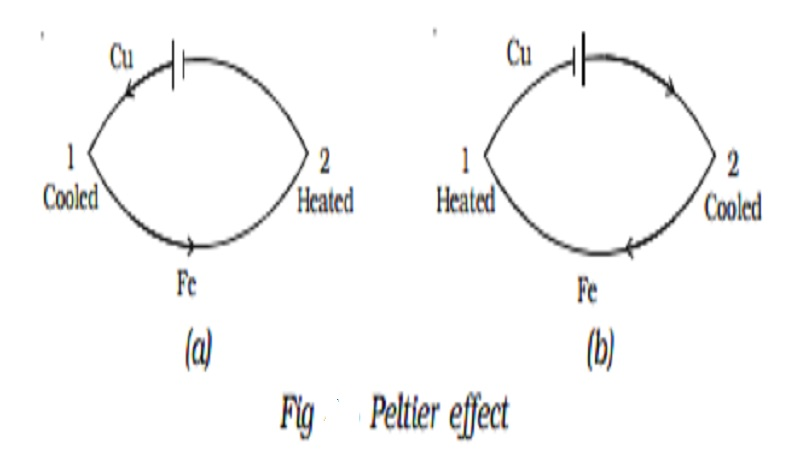Home | | Physics | | Physics | Peltier effect and Peltier Co-efficient (π)

Peltier effect and Peltier Co-efficient (π)Peltier effect : In 1834, a French scientist Peltier discovered that when electric current is passed through a circuit consisting of two dissimilar metals, heat is evolved at one junction and absorbed at the other junction. This is called Peltier effect. Peltier effect is the converse of Seebeck effect.

Peltier effect

In 1834, a French scientist Peltier discovered that when electric current is passed through a circuit consisting of two dissimilar metals, heat is evolved at one junction and absorbed at the other junction. This is called Peltier effect. Peltier effect is the converse of Seebeck effect.In a Cu-Fe thermocouple, at the junction 1 (Fig 3.4a) where the current flows from Cu to Fe, heat is absorbed (so, it gets cooled) and at the junction 2 where the current flows from Fe to Cu heat is liberated (so, it gets heated). When the direction of the current is reversed (Fig 3.4b) junction 1 gets heated and the junction 2 gets cooled. Hence Peltier effect is reversible.

Peltier Co-efficient (π)

The amount of heat energy absorbed or evolved at one of the junctions of a thermocouple when one ampere current flows for one second (one coulomb) is called Peltier coefficient. It is denoted by π. Its unit is volt. If H is the quantity of heat absorbed or evolved at one junction then H = π It

The Peltier coefficient at a junction is the Peltier emf at that junction. The Peltier coefficient depends on the pair of metals in contact and the temperature of the junction.

Study Material, Lecturing Notes, Assignment, Reference, Wiki description explanation, brief detail

Related Topics